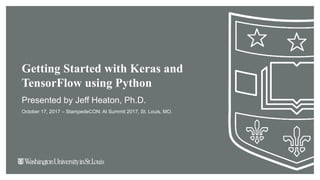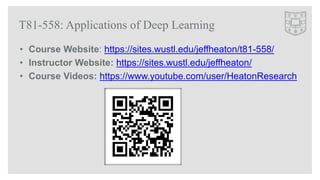Successfully reported this slideshow.

# Getting Started with Keras and TensorFlow - StampedeCon AI Summit 2017×

# Getting Started with Keras and TensorFlow - StampedeCon AI Summit 2017

This technical session provides a hands-on introduction to TensorFlow using Keras in the Python programming language. TensorFlow is Google’s scalable, distributed, GPU-powered compute graph engine that machine learning practitioners used for deep learning. Keras provides a Python-based API that makes it easy to create well-known types of neural networks in TensorFlow. Deep learning is a group of exciting new technologies for neural networks. Through a combination of advanced training techniques and neural network architectural components, it is now possible to train neural networks of much greater complexity. Deep learning allows a model to learn hierarchies of information in a way that is similar to the function of the human brain.

This technical session provides a hands-on introduction to TensorFlow using Keras in the Python programming language. TensorFlow is Google’s scalable, distributed, GPU-powered compute graph engine that machine learning practitioners used for deep learning. Keras provides a Python-based API that makes it easy to create well-known types of neural networks in TensorFlow. Deep learning is a group of exciting new technologies for neural networks. Through a combination of advanced training techniques and neural network architectural components, it is now possible to train neural networks of much greater complexity. Deep learning allows a model to learn hierarchies of information in a way that is similar to the function of the human brain.

### Getting Started with Keras and TensorFlow - StampedeCon AI Summit 2017

1. 1. Getting Started with Keras and TensorFlow using Python Presented by Jeff Heaton, Ph.D. October 17, 2017 – StampedeCON: AI Summit 2017, St. Louis, MO.
2. 2. Jeff Heaton, Ph.D. • Lead Data Scientist at RGA • Adjunct Instructor at Washington University • Fellow of the Life Management Institute (FLMI) • Senior Member of IEEE • Kaggler (Expert level) • http://www.jeffheaton.com – (contact info at my website)
3. 3. T81-558: Applications of Deep Learning • Course Website: https://sites.wustl.edu/jeffheaton/t81-558/ • Instructor Website: https://sites.wustl.edu/jeffheaton/ • Course Videos: https://www.youtube.com/user/HeatonResearch
4. 4. Presentation Outline • Deep Learning Framework Landscape • TensorFlow as a Compute Graph/Engine • Keras and TensorFlow • Keras: Classification • Keras: Regression • Keras: Computer Vision and CNN • Keras: Time Series and RNN • GPU
5. 5. Deep Learning Framework Landscape (discontinued)
6. 6. Deep Learning Mindshare on GitHub
7. 7. What are Tensors? Why are they flowing? • Tensor of Rank 0 (or scaler) – simple variable • Tensor of Rank 1 (or vector) – array/list • Tensor of Rank 2 (or matrix) – 2D array • Tensor of Rank 3 (or cube) – 3D array • Tensor of Rank 4 (tesseract/hypercube) – 4D array • Higher ranks (hypercube) – nD array
8. 8. TensorFlow as a Compute Graph/Engine
9. 9. What are Tensors? Why are they flowing? • Tensor of Rank 0 (or scaler) – simple variable • Tensor of Rank 1 (or vector) – array/list • Tensor of Rank 2 (or matrix) – 2D array • Tensor of Rank 3 (or cube) – 3D array • Tensor of Rank 4 (tesseract/hypercube) – 4D array • Higher ranks (hypercube) – nD array
10. 10. What is a Computation Graph? import tensorflow as tf matrix1 = tf.constant([[3., 3.]]) matrix2 = tf.constant([[2.],[2.]]) product = tf.matmul(matrix1, matrix2) with tf.Session() as sess: result = sess.run([product]) print(result)
11. 11. Computation Graph with Variables import tensorflow as tf sess = tf.InteractiveSession() x = tf.Variable([1.0, 2.0]) a = tf.constant([3.0, 3.0]) x.initializer.run() sub = tf.subtract(x, a) print(sub.eval()) # ==> [-2. -1.] sess.run(x.assign([4.0, 6.0])) print(sub.eval()) # ==> [1. 3.]
12. 12. Computation Graph for Mandelbrot Set
13. 13. Mandelbrot Set Review • Some point c is a complex number with x as the real part, y as the imaginary part. • z0 = 0 • z1 = c • z2 = z1 2 + c • ... • zn+1 = zn 2 + c
14. 14. Mandelbrot Rendering in TensorFlow xs = tf.constant(Z.astype(np.complex64)) zs = tf.Variable(xs) ns = tf.Variable(tf.zeros_like(xs, tf.float32)) tf.global_variables_initializer().run() # Compute the new values of z: z^2 + x zs_ = zs*zs + xs # Have we diverged with this new value? not_diverged = tf.abs(zs_) < 4 step = tf.group( zs.assign(zs_), ns.assign_add(tf.cast(not_diverged, tf.float32)) ) for i in range(200): step.run()
15. 15. Keras and TensorFlow
16. 16. Tools Used in this Presentation • Anaconda Python 3.6 • Google TensorFlow 1.2 • Keras 2.0.6 • Scikit-Learn • Jupyter Notebooks
17. 17. Installing These Tools • Install Anaconda Python 3.6 • Then run the following: – conda install scipy – pip install sklearn – pip install pandas – pip install pandas-datareader – pip install matplotlib – pip install pillow – pip install requests – pip install h5py – pip install tensorflow==1.2.1 – pip install keras==2.0.6
18. 18. Keras and TensorFlow Keras TF Learn TensorFlow Compute Graphs CPU GPU Theano Compute Graphs MXNet Compute Graphs
19. 19. Anatomy of a Neural Network • Input Layer – Maps inputs to the neural network. • Hidden Layer(s) – Helps form prediction. • Output Layer – Provides prediction based on inputs. • Context Layer – Holds state between calls to the neural network for predictions.
20. 20. • Deep learning is almost always applied to neural networks. • A deep neural network has more than 2 hidden layers. • Deep neural networks have existed as long as traditional neural networks. – We just did not have a way to train deep neural networks. – Hinton (et al.) introduced a means to train deep belief neural networks in 2006. • Neural networks have risen three times and fallen twice in their history. Currently, they are on the rise. What is Deep Learning
21. 21. The True Believers – Luminaries of Deep Learning • From left to right: • Yann LeCun • Geoffrey Hinton • Yoshua Bengio • Andrew Ng
22. 22. • Deep neural networks often accomplish the same task as other models, such as: – Support Vector Machines – Random Forests – Gradient Boosted Machines • For many problems deep learning will give a less accurate answer than the other models. • However, for certain problems, deep neural networks perform considerably better than other models. Why Use Deep Learning
23. 23. Why Deep Learning (high y-axis is good)
24. 24. Supervised or Unsupervised? Supervised Machine Learning • Usually classification or regression. • For an input, the correct output is provided. • Examples of supervised learning: – Propensity to buy – Credit scoring Unsupervised Machine Learning • Usually clustering. • Inputs analyzed without any specification of a correct output. • Examples of unsupervised learning: – Clustering – Dimension reduction
25. 25. Types of Machine Learning Algorithm • Clustering: Group records together that have similar field values. For example, customers with common attributes in a propensity to buy model. • Regression: Learn to predict a numeric outcome field, based on all of the other fields present in each record. For example, predict the amount of coverage a potential customer might buy. • Classification: Learn to predict a non-numeric outcome field. For example, learn the type of policy an existing customer has a potential of buying next.
26. 26. Problems that Deep Learning is Well Suited to
27. 27. Keras: Classification
28. 28. • Classic classification problem. • 150 rows with 4 predictor columns. • All 150 rows are labeled as a species of iris. • Three different iris species. • Created by Sir Ronald Fisher in 1936. • Predictors: – Petal length – Petal width – Sepal length – Sepal width The Classic Iris Dataset
29. 29. The Classic Iris Dataset sepal_length sepal_width petal_length petal_width class 5.1 3.5 1.4 0.2 Iris-setosa 7.0 3.2 4.7 1.4 Iris-versico 6.3 3.3 6.0 2.5 Iris-virginica 6.4 3.2 4.5 1.5 Iris-versicolor 5.8 2.7 5.1 1.9 Iris-virginica 4.9 3.0 1.4 0.2 Iris-setosa … … … … …
30. 30. Are the Iris Data Predictive?
31. 31. Keras Classification: Load and Train/Test Split path = "./data/" filename = os.path.join(path,"iris.csv") df = pd.read_csv(filename,na_values=['NA','?']) species = encode_text_index(df,"species") x,y = to_xy(df,"species") # Split into train/test x_train, x_test, y_train, y_test = train_test_split( x, y, test_size=0.25, random_state=42)
32. 32. Keras Classification: Build NN and Fit model = Sequential() model.add(Dense(10, input_dim=x.shape, kernel_initializer='normal', activation='relu')) model.add(Dense(1, kernel_initializer='normal')) model.add(Dense(y.shape,activation='softmax')) model.compile(loss='categorical_crossentropy', optimizer='adam') monitor = EarlyStopping(monitor='val_loss', min_delta=1e-3, patience=5, verbose=1, mode='auto') model.fit(x,y,validation_data=(x_test,y_test),callbacks=[monitor],ve rbose=2,epochs=1000)
33. 33. Keras Classification: Build NN and Fit # Evaluate success using accuracy # raw probabilities to chosen class (highest probability) pred = model.predict(x_test) pred = np.argmax(pred,axis=1) y_compare = np.argmax(y_test,axis=1) score = metrics.accuracy_score(y_compare, pred) print("Accuracy score: {}".format(score))
34. 34. Keras: Regression
35. 35. • Classic regression problem. • Target: mpg • Predictors: – cylinders – displacement – horsepower – weight – acceleration – year – origin – name Predict a Car’s Miles Per Gallon (MPG)
36. 36. Predict a Car’s Miles Per Gallon (MPG) mpg cylinders displace ment horsepow er weight accelerati on year origin name 18 8 307 130 3504 12 70 1 chevrolet chevelle malibu 15 8 350 165 3693 11.5 70 1 buick skylark 320 18 8 318 150 3436 11 70 1 plymouth satellite 16 8 304 150 3433 12 70 1 amc rebel sst 17 8 302 140 3449 10.5 70 1 ford torino 15 8 429 198 4341 10 70 1 ford galaxie 500 14 8 454 220 4354 9 70 1 chevrolet impala
37. 37. • Models such as GBM or Neural Network can predict the MPG to around +/-2.7 accuracy. • Result of regression can be given in equation form (though not as accurate as a model): Regression Models - MPG
38. 38. Keras Regression: Load and Train/Test Split path = "./data/" filename_read = os.path.join(path,"auto-mpg.csv") df = pd.read_csv(filename_read,na_values=['NA','?']) cars = df['name'] df.drop('name',1,inplace=True) missing_median(df, 'horsepower') x,y = to_xy(df,"mpg")
39. 39. Keras Regression: Build and Fit model = Sequential() model.add(Dense(10, input_dim=x.shape, kernel_initializer='normal', activation='relu')) model.add(Dense(1, kernel_initializer='normal')) model.compile(loss='mean_squared_error', optimizer='adam') monitor = EarlyStopping(monitor='val_loss', min_delta=1e-3, patience=5, verbose=1, mode='auto') model.fit(x,y,validation_data=(x_test,y_test),callbacks=[monitor],ve rbose=2,epochs=1000)
40. 40. Keras Regression: Predict and Evaluate # Predict pred = model.predict(x_test) # Measure RMSE error. RMSE is common for regression. score = np.sqrt(metrics.mean_squared_error(pred,y_test)) print("Final score (RMSE): {}".format(score))
41. 41. • The iris and MPG datasets are nicely formatted. • Real world data is a complex mix of XML, JSON, textual formats, binary formats, and web service accessed content (the variety V in “Big Data”). • More complex security data will be presented later in this talk. Preparing Data for Predictive Modeling is Hard
42. 42. Keras: Computer Vision and CNN
43. 43. • We will usually use classification, though regression is still an option. • The input to the neural network is now 3D (height, width, color). • Data are not transformed, no zscores or dummy variables. • Processing time is usually much longer. • We now have different layer types: dense layers (just like before), convolution layers and max pooling layers. • Data will no longer arrive as CSV files. TensorFlow provides some utilities for going directly from image to the input of a neural network. Predicting Images: What is Different?
44. 44. Sources of Image Data: CIFAR10 and CIFAR100
45. 45. Sources of Image Data: ImageNet
46. 46. Sources of Training Data: The MNIST Data Set
47. 47. Recognizing Digits
48. 48. Convolutional Neural Networks (CNN) A LeNET-5/CNN Network (LeCun, 1998) Dense Layers - Fully connected layers. Convolution Layers - Used to scan across images. Max Pooling Layers - Used to downsample images. Dropout Layer - Used to add regularization.
49. 49. Loading the Digits (x_train, y_train), (x_test, y_test) = mnist.load_data() print("Shape of x_train: {}".format(x_train.shape)) print("Shape of y_train: {}".format(y_train.shape)) print() print("Shape of x_test: {}".format(x_test.shape)) print("Shape of y_test: {}".format(y_test.shape)) Shape of x_train: (60000, 28, 28) Shape of y_train: (60000,) Shape of x_test: (10000, 28, 28) Shape of y_test: (10000,)
50. 50. Display a Digit %matplotlib inline import matplotlib.pyplot as plt import numpy as np digit = 101 # Change to choose new digit a = x_train[digit] plt.imshow(a, cmap='gray', interpolation='nearest') print("Image (#{}): Which is digit '{}'". format(digit,y_train[digit]))